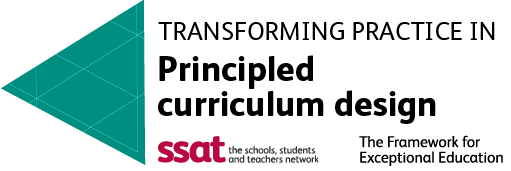#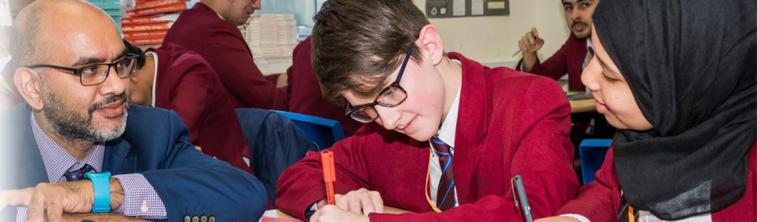#### Useful Resources and Revision Support

• https://sparxmaths.uk/ is our online homework tool as well as a powerful revision resource. All students will have weekly homework set that is bespoke to them. It will be set at a challenging but achievable level. All students should aim for 100% and must watch the video for any question they get wrong. Relevant revision clip numbers can be found within students' exercise books in the PLCs.

• Recommended Maths Books:

https://corbettmaths.com/ has videos, exam style questions and textbook exercises for all topics up to GCSE level. It also has ‘5-a-day’ worksheets that are excellent for revision.

https://mrcartermaths.com/ has differentiated exercises with randomly generated numbers that help with repeated practice. It is currently mostly focused on topics up to GCSE level but it has started to have some A-level content too. Students need to ask their teacher for the login.

https://mathsgenie.co.uk/ is another website with exam-style questions and easy access to past papers

## Mathematics is vital for everyday life and in understanding the world around us. It is not only essential to science, technology and engineering but is applied to many other subjects for example the use of statistics.

Our vision is to ensure that all pupils develop an appreciation of mathematics and have the best possible mathematics education to maximise their future opportunities. We have high expectations for all and set challenging targets for all pupils.

In Key Stage 3 our focus is to embed an enjoyment of the subject, develop creative problem solving, as well as increasing confidence and fluency in using mathematical skills. We follow a maths mastery approach and use the White Rose Maths scheme as the basis for our teaching. As students progress, we have a range of pathways to both support and challenge all students. From September 2023, all students in Year 10 will sit the Statistics GCSE. As well as the Mathematics GCSE in Year 11 (Edexcel) the most able students also sit Level 2 Further Mathematics (AQA) in Year 11 in preparation for sixth form study.

In addition, we offer enrichment opportunities outside the curriculum to enhance pupils’ enjoyment of mathematics such as after school clubs, external visits and the UK Maths Challenge. All students have access to SparxMaths, which is used for homework but is also expected to be used for independent study. It has very specific videos that clearly demonstrate the skills needed and allows students to test and track their understanding.

Our ethos involves working with all learners to be the best they can be and faculty teachers working together as a team to achieve the best for our students. We greatly value the support that parents and carers give to our students and welcome all feedback.

### AssessmentPearson Edexcel GCSEs Mathematics (9-1)

Specification and papers can be found using the link below.

https://qualifications.pearson.com/en/qualifications/edexcel-gcses/mathematics-2015.html >>

### Enrichment

The mathematics department has an extensive enrichment programme. Outside of lessons we enter students from all year groups into the national UKMT maths challenge. In addition to this, girls in the 6th form can enter the Maths Olympiad for Girls (MOG).  Year 10 students get the opportunity to attend the Advanced Maths Support Programme Maths Feast event. Whilst Year 7 and Year 8 have a weekly after school maths club that is well attended. We offer homework support afterschool with maths teacher assistance. We have a maths challenge within school as part of the house competition system where all year groups can enter to compete for their house. Inside the classroom we offer the top sets in Year 11 the opportunity to study a Level 2 Further Maths qualification alongside their GCSE studies as well as having a strong uptake of Level 3 Core Maths and A-level Further Mathematics in sixth form. Weekly revision for Year 11 and A level students is offered from October half term onwards with Statistics GCSE sessions to follow later in the year.

### Curriculum Overview

#### Year 7

Term 1:

• Sequences
• Algebraic Notation
• Equality and Equivalence
• Place Value
• Fractions, Decimals and Percentages

Term 2:

• Applications with Number (including Area and Perimeter)
• Fractions and Percentages of Amounts
• Working with Directed Number

Term 3:

• Constructing and Measuring Angles
• Geometric Reasoning with Angles
• Developing Number Sense
• Sets and Probability
• Prime Numbers and Proof

#### Year 8

Term 1:

Ratio and Scale

• Multiplicative Change
• Multiplying and Dividing Fractions
• Coordinates and Linear Equations
• Representing Data

Term 2:

• Brackets, Equations and Inequalities
• Sequences and Indices
• Fractions and Percentages
• Standard Index Form

Term 3:

• Angles in Parallel Lines and Polygons
• Area of Trapezia and Circles
• The Data Handling Cycle
• Averages

#### Year 9

Term 1:

• Straight Line Graphs
• Forming and Solving Equations
• Testing Conjectures
• Volume and Surface Area
• Constructions and Congruency

Term 2 :

• Fractions, Decimals and Percentages
• Percentage Change
• Maths and Money
• Geometric Reasoning With Angles
• Reflection, Rotation and Translation

Term 3:

• Pythagoras Theorem
• Enlargement and Similarity
• Ratio
• Probability

#### Year 10

Year  10 Statistics: From September 2023, all students in Year 10 will sit the Statistics GCSE.

Foundation:

• Number sense, Indices, factors and multiples
• Percentages, decimals and ratio
• Fractions and money problems
• Place value, estimating, error intervals and standard form
• Expanding, simplifying, factorising algebra
• Sequences, solving and rearranging algebra
• Substitution, inequalities and simultaneous equations
• Straight line Graphs and Quadratic Graphs
• Angle facts, plans and elevations, nets
• Transformations, Similarity and Congruence
• Area and perimeter of 2D shapes
• Volume and Surface Area of prisms
• Compound Units
• Pythagoras Theorem
• Column Vectors
• Averages
• Bar charts, Pictograms, Scatter graphs, Time series, Pie charts and stem and leaf diagrams
• Probability

Year 10 Crossover:

• Indices, factors and multiples
• Percentages, decimals and ratio
• Fractions and money problems
• Estimating, error intervals, upper and lower bounds and standard form
• Surds
• Direct and Inverse Proportion
• Expanding, simplifying, factorising algebra
• Sequences, solving and rearranging algebra
• Substitution, inequalities and simultaneous equations
• Straight line Graphs and Quadratic Graphs
• Angle facts, angles in polygons, circle theorems
• Transformations, Similarity and Congruence
• Area and perimeter of 2D shapes
• Volume and Surface Area of 3D shapes
• Compound Units
• Pythagoras Theorem
• Right angled Trigonometry
• Sine and Cosine rule
• Column Vectors
• Algebraic Vectors
• Averages
• Scatter graphs, Time series, Pie charts, Frequency Polygons, Cumulative Frequency Graphs, Box Plots
• Probability trees, Venn diagrams

Year 10 Higher:
• Indices, factors and multiples
• Percentages, decimals and ratio
• Fractions and money problems
• Estimating, error intervals, upper and lower bounds and standard form
• Surds
• Direct and Inverse Proportion
• Expanding, simplifying, factorising algebra
• Sequences, solving and rearranging algebra
• Iteration
• Substitution, inequalities and simultaneous equations
• Straight line Graphs and Quadratic Graphs
• Graphical Inequalities
• Completing the Square
• Equation of a circle
• Equation of a tangent of a circle
• Angle facts, angles in polygons, circle theorems
• Bearings
• Transformations, Similarity and Congruence
• Area and perimeter of 2D shapes
• Area and perimeter of sectors
• Volume and Surface Area of 3D shapes
• Compound Units
• Pythagoras Theorem
• Right angled Trigonometry
• Sine and Cosine rule
• Column Vectors
• Algebraic Vectors
• Averages
• Scatter graphs, Time series, Pie charts, Frequency Polygons, Cumulative Frequency Graphs, Box Plots, Histograms
• Probability trees, Venn diagrams
• Conditional Probability

#### Year 11

Year 11 will spend time revisiting and furthering work completed in Year 10 with the following topics introduced:
Foundation:
• Laws of indices: fractional and negative
• Direct and inverse proportion
• Upper and lower bounds
• Geometric and Fibonacci Sequences
• Cubic and reciprocal graphs
• Constructions and loci
• Bearings
• Volume and surface area of other 3D shapes
• Right angled trigonometry
• Frequency Polygons
• Frequency Trees
• Probability Trees
• Venn Diagrams

Higher:
• Algebraic fractions
• Algebraic proof
• Function notation, inverse functions, composite functions
• Graph transformations
• Estimating the gradient of a tangent
• Estimating the area under a curve
• Constructions and Loci
• Capture-recapture

In addition to GCSE maths, higher ability students in year 11 also have the opportunity to sit Further Maths Level 2. The following topics are covered in this course:
1. Number
2. Algebra
3. Coordinate Geometry
4. Calculus
5. Matrix Transformations
6. Geometry

Student Support and Wellbeing: Worried about something?

Report it.

•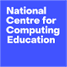•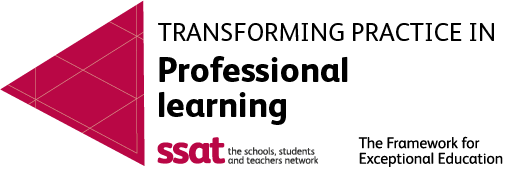•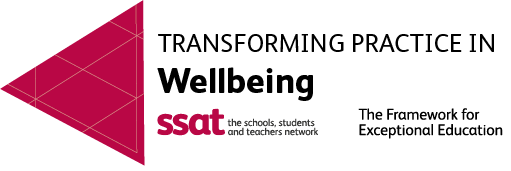•••••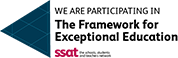•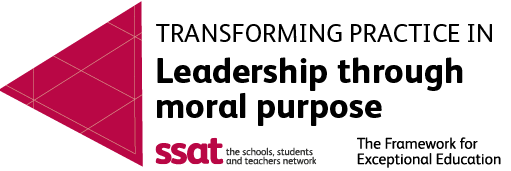•